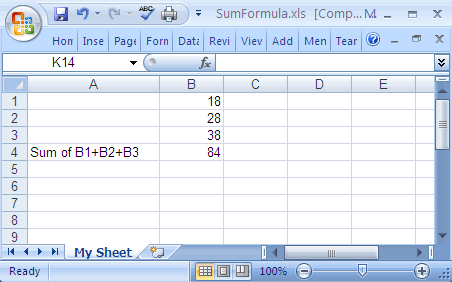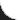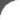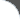Home | Tutorials | Articles | Videos | Products | Tools | Search
 Interviews | Open Source | Tag Cloud | Follow Us | Bookmark | ContactIn Browser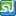StumbleUpon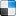del.icio.us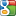Google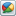Google Buzz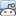reddit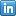LinkedIn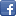Facebook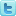TwitterLinkedin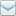E-Mail
 Excel > JExcel API > How to add Sum Formula to Excel Spreadsheet

# How to add Sum Formula to Excel Spreadsheet

Java Excel API is an open source java library to read, write and modify Excel spread sheets. This requires the library jxl-2.6.12.jar to be in classpath. The following example shows how to add sum formula to a Cell in Excel Spreadsheet.

 File Name  : com/bethecoder/tutorials/jexcelapi/write/SumFormulaTest.java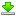Author  : Sudhakar KV Email  : kven[email protected]

 ``` package com.bethecoder.tutorials.jexcelapi.write; import java.io.File; import java.io.IOException; import jxl.Workbook; import jxl.read.biff.BiffException; import jxl.write.Formula; import jxl.write.Label; import jxl.write.Number; import jxl.write.WritableSheet; import jxl.write.WritableWorkbook; import jxl.write.WriteException; public class SumFormulaTest {   /**    * @param args    * @throws IOException     * @throws IOException     * @throws WriteException     * @throws BiffException     */   public static void main(String[] args) throws IOException, WriteException {     //Creates a writable workbook with the given file name     WritableWorkbook workbook = Workbook.createWorkbook(new File("C:/JXL/SumFormula.xls"));     WritableSheet sheet = workbook.createSheet("My Sheet", 0);     Number numCell = new Number(1, 0, 18);     sheet.addCell(numCell);     Number numCell2 = new Number(1, 1, 28);     sheet.addCell(numCell2);          Number numCell3 = new Number(1, 2, 38);     sheet.addCell(numCell3);          //Create a formula for adding cells     Formula sum = new Formula(1, 3, "B1+B2+B3");     sheet.addCell(sum);          //Create label for sum     Label sumLabel = new Label(0, 3, "Sum of B1+B2+B3");     sheet.addCell(sumLabel);          //Writes out the data held in this workbook in Excel format     workbook.write();      //Close and free allocated memory      workbook.close();    } }```

It gives the following output,